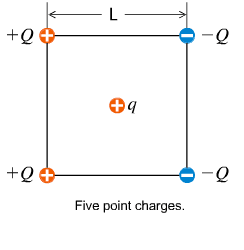# Simple electric force problem with point masses includes picture

• 1901phoenix

#### 1901phoenix

Simple electric force problem with point masses... includes picture!

## Homework Statement

Four point charges of +/- q are arranged on the corners of a square of side L as illustrated in the figure. What is the net electric force that these charges exert on a point charge q placed at the center of the square (in terms of Q, q, L, and the Coulomb constant, K)

Diagram:## Homework Equations

Coulomb's law: F= k * (q * q)/r^2

## The Attempt at a Solution

I started off my drawing a free body diagram. Picking one Q (the lower left) I drew one electrostatic force (from the upper Q) going vertically downwards because they are like charges so they have to repel.
I drew another electrostatic force (from the Q to the right, with the negative charge) going to the right in the x direction.
There is also going to be a force from Q on q in the center.

From here I am totally confused. I think if I am able to resolve all of the forces for one of the Q's I can use symmetry to find my answer but I am not sure how. I am also wary of how to relate any of the Q's to q. I hope this isn't too confusing for anyone, I really appreciate it.

Why do you need the forces of the charges on the square upon each other?

I am not sure... should I just take into account the forces applied by each of the corners on the center, q.

I can draw a free body diagram for that, but without concrete number I am lost on how to write the expression.

I am not sure... should I just take into account the forces applied by each of the corners on the center, q.

Yes, the question asks for the net force on the charge at the center, so concentrate your efforts there.

I can draw a free body diagram for that, but without concrete number I am lost on how to write the expression.

Well, variables are just numbers in disguise! I'm afraid you'll have to suffer the algebra. If you look carefully at the diagram, before you even write down a single equation, you might be able to spot some symmetries that will simplify things considerably. Pay attention to the placement and polarities of the charges.